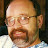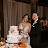Exponents 4 Power of a Product – Tutor AlgebraExponents 4 Power of a Product – Tutor Algebra

This math video tutors and gives instruction about exponents. The instruction is a brief and to the point teaching lesson on how to learn algebra and manipulate variables.
Exponents are a simple concept to start but can be very complicated when used with several operations. Powers raised to powers, negative exponents, and fraction exponents are some of the topics covered in this series of videos.

algebra tutors,algebra,college,cube,educational,equation,exponent,expression,for,ged,help,high,homework,how to,inequality,instruction,learn,lesson,linear,math,mathematics,online,practice,pre,property,pythagorean,root,school,simplify,solve,solving,square,system,teach,theorem,tutor,tutorial,tutoring,variable,video,with

algebratutors.org

1.Paul Bogdan says:
2.Austin says: